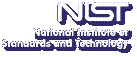Up# Adiabatic Optimization and the Fundamental Gap Theorem

Michael Jarret
Department of Physics, University of Maryland

Tuesday, May 27, 2014 15:00-16:00,
Building 225, Room B111
Gaithersburg
Tuesday, May 27, 2014 13:00-14:00,
Room 1-4058
Boulder

Abstract:

By the adiabatic theorem, the runtime of an adiabatic optimization algorithm is upper bounded by $O(1/\gamma^3)$, where $\gamma$ is the minimal eigenvalue gap of a corresponding Hamiltonian. Thus, analyzing the complexity of adiabatic algorithms reduces to bounding $\gamma$. Typically, this is a very difficult problem. In this work, rather than directly bounding gaps of Hamiltonians proposed for adiabatic algorithms, we begin to map the boundary between small- and large-gapped Hamiltonians. Our tools may be of independent interest in areas such as condensed-matter physics and spectral graph theory.

The fundamental gap conjecture (FGC) proposes a tight lower bound of $3\pi/D^2$ to the difference between the two lowest Dirichlet eigenvalues (the gap) of a Schrödinger operator $−\nabla^2+V(x)$ with convex potential $V$ on a compact convex domain $\Omega \subset R^n$ of diameter $D$. In a recent breakthrough, Andrews and Clutterbuck proved this longstanding conjecture. Here, we combine tools drawn from the FGC literature and the quantum information literature to prove some discrete analogues of the FGC and provide tight bounds for certain path and hypercube graphs. We also provide a counterexample to an original intuition behind adiabatic optimization algorithms: that since quantum optimization algorithms sometimes tunnel out of local minima that trap simulated annealing, a single local minimum implies a large gap.

Speaker Bio: Michael Jarret is a PhD candidate in physics at the University of Maryland. Michael studies quantum information with Stephen Jordan and has a BS in physics and mathematics from SUNY Binghamton.

Contact: S. Jordan

Note: Visitors from outside NIST must contact Cathy Graham; (301) 975-3800; at least 24 hours in advance.